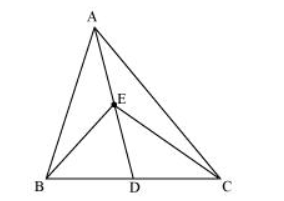# In the given figure, E is any point on median AD of a ΔABC

Question. In the given figure, $E$ is any point on median $A D$ of a $\triangle A B C$. Show that $\operatorname{ar}(A B E)=\operatorname{ar}(A C E)$Solution:

AD is the median of ΔABC. Therefore, it will divide ΔABC into two triangles of equal areas.

$\therefore$ Area $(\triangle \mathrm{ABD})=$ Area $(\triangle \mathrm{ACD}) \ldots(1)$

ED is the median of ΔEBC.

$\therefore$ Area $(\Delta \mathrm{EBD})=$ Area $(\Delta \mathrm{ECD}) \ldots(2)$

On subtracting equation (2) from equation (1), we obtain

Area $(\triangle A B D)$ - Area $(E B D)=$ Area $(\triangle A C D)-$ Area $(\triangle E C D)$

Area $(\triangle \mathrm{ABE})=\operatorname{Area}(\triangle \mathrm{ACE})$